# 3D and perspective techniques

I’ve had a semester of technical drawing where we learned a few things some might find useful when drawing 3d renditions. Most people can eyeball these things, but some, like me, need to draw it up in a more precise way.
I use nanoCAD, which is freeware. It has some annoying glitches, but all in all it is great for simple 2d drawing.
First example is the well known perspective of a block seen looking directly at a corner.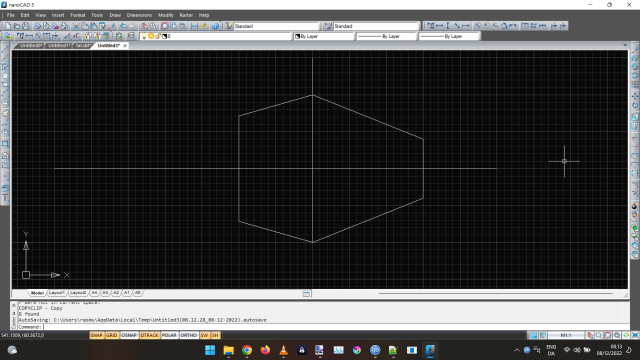I’ve made it a bit off center and asymmetrical to illustrate things better.
The way to make sure things are in scale, you choose a point to measure from, typically the corner closest to you, the center line in my example. Here the center line is 4 5x5 blocks high.
To measure a distance disappearing into the left, I use a base line, and measure along with that. In the example, I’ve measured 4 blocks down. I then draw a line from the far right point, the “point of disappearing”. Where this line crosses my line from the left is where a point will be equal to the height of the block.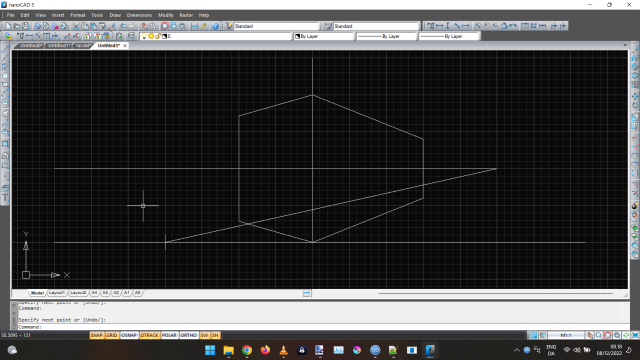If I do this for both sides, I’ll get this perspective rendition of a cube. On the picture, I’ve circled the intersections.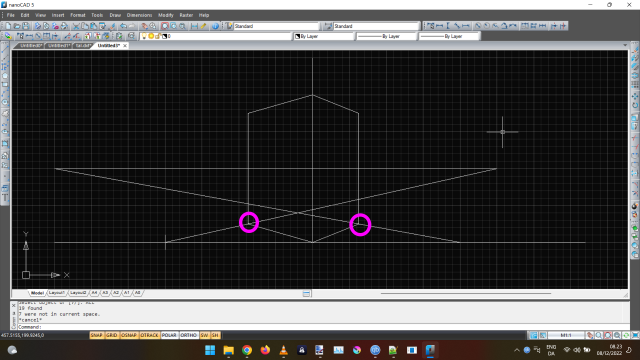This is an idea of what it would look like from above with the line of sight drawn in. Not a precise picture of it though.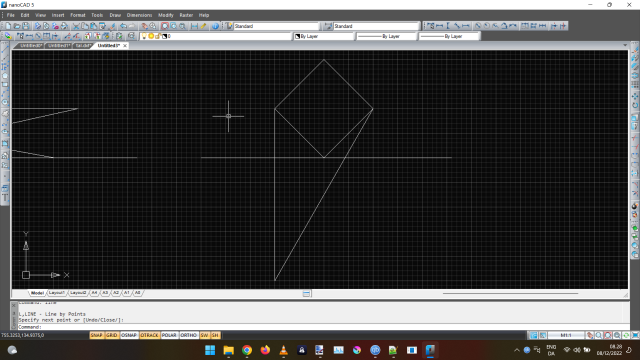To measure vertically is easy, simply mark the point on the center line and draw the perspective lines. In the picture I’ve drawn a “window” 1x1 blocks, circled in pink.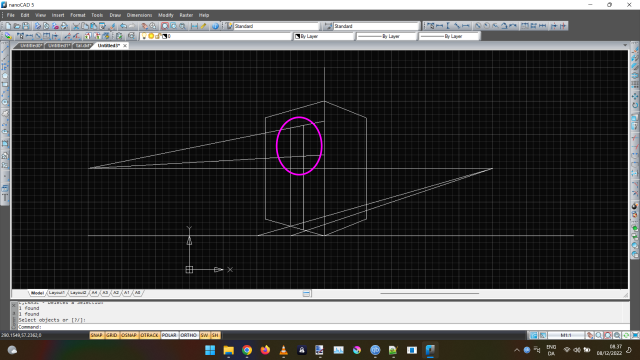2 Likes

I used this method to draw the outline for a simple dot matrix digit.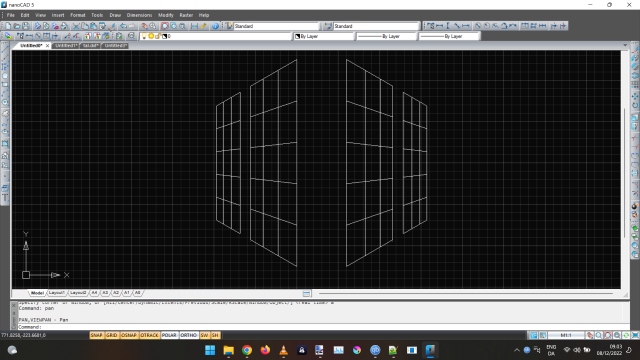2 Likes

The second method is more cumbersome. It’s a lot better when you can use script/programming to generate the coordinates. But here it is anyway.
You imagine yourself standing in a spot, looking at an object, a cube in my example.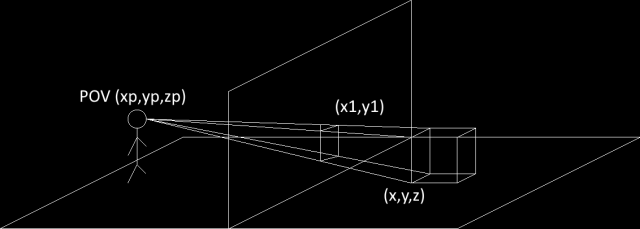Between yourself and the object there is a plane, the drawing surface, your view. The cube has real life coordinates in space, (x,y,z). Each point correlates to a point on the drawing surface, (x1,y1). This point is where your line of sight crosses the drawing plane. Finding this point is a question of geometry.
If you set your POV to (0,0,0), and your height (z) is 7 (in whatever scale you’re using), the coordinates to the bottom, left, front corner (as seen from pov) are
(30, 5, -7) (my cube is 5x5x5)

In this example, x is the direction you’re looking in, right on the drawing. Y is to sides, positive into the drawing, and z is the height.
The drawing plane has an x value of 20.

The (x1,y1) coordinates are oriented differently, x1 is out of the drawing, y1 is up, as in a normal xy coordinate system, if seen from the pov. (0,0) is the spot directly in front.

The corner is 30 “away” (x value), the plane 20. The corner is 5 to the side (y direction). So the x1 coordinate is
`5*20/30 = 3.3333`
The corner is 7 “down” (z value -7). So the y1 coordinate is
`-7*20/30 = -4.6667`

I’ve shown the example as seen when looking down on it, and in from the side here.

The 6 visible corners of the cube would have the coordinates

bottom left front
(x,y,z) = (30,5,-7)
(x1,y1) = (3.3333,-4.6667)

bottom right front
(x,y,z) = (30,0,-7)
(x1,y1) = (0,-4.6667)

top left front
(x,y,z) = (30,5,-2)
(x1,y1) = (3.3333,-1.3333)

top right front
(x,y,z) = (35,0,-2)
(x1,y1) = (0,-1.3333)

top left back
(x,y,z) = (30,5,-2)
(x1,y1) = (2.8571,-1.1429)

top right back
(x,y,z) = (35,0,-2)
(x1,y1) = (0,-1.1429)

The corners in back are 5 further away, so instead of multiplying by 20 (distance to the plane) and dividing with 30 (distance to corner), you divide with 35.

When drawn, this will look like so

2 Likes

Isometrics is kinda my new jam these days (you should check them out), and I have been able to be very precise using illustrator to draw my “3D” elements. I have a few watches that I have made and I am currently experimenting with all kinds of ways to make more of a variety of digits. Honestly I hope to get into a real 3d work environment app in the future though.

3 Likes

Yes Yes Yes . This is what this community is about. I see Ramus Blocks rotating and flipping the digit for the reveal. The work has been done with the moon phase sliders and masks. I tried a flipping numerals but had to give up. I got to 2 I think. Brain will not let me go there.

2 Likes

I’ve seen some of those, very cool. My main interest is in the geeky, techy stuff, so not really interested in programs that’ll do it for me. In my eyes, that’s just no fun.

But isometrics aren’t perspective, I do like the 3d feel.

I’ve made some javascripts that can do the math involved here, enter the x,y,z coordinates and it draws the the point, line or whatever. If there is any interest in this, I’ll write a script here that returns the x1,y1 coordinates for any given x,y,z.

2 Likes

Well just to clarify, it doesn’t do it for me. I have to draw everything on an isometric grid. As far as perspective, right now I have everything aligned at 30 or -30 degrees to get that overhead perspective. But there’s no reason why you couldn’t pick a vanishing point and draw everything according too that. But yeah, not a lot of actual math with my method.

2 Likes

The first method doesn’t involve any math at all, it is a fairly simple drawing technique. To draw a rendition, you’d need two copies of your pic, and then draw an awful lot of guidelines.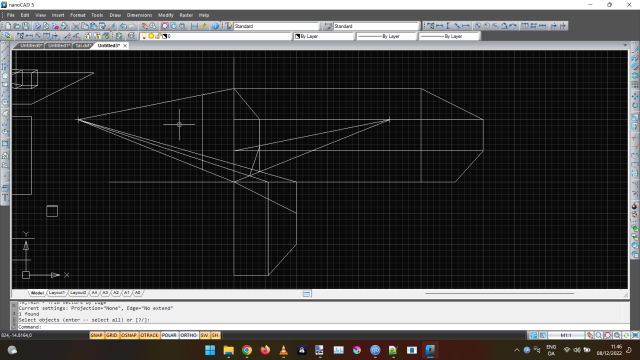made a few mistakes on my first drawing, corrected them.

2 Likes

Very good topic. much appreciated

2 Likes

I’m thinking of making an analog watchface with the face bent in the middle. Sort of the way Dali would have painted it, if he’d been an engineer and not an artist.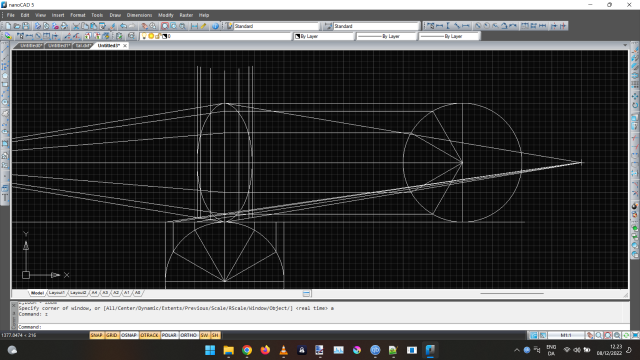The watchhands would then turn the corner when they rotate past the center.
Numerous problems in this, as seen in the picture, the perspective would have to be very wide for the face to be more than a sliver. And the wider the perspective, the less the effect.
I think that can be fixed with using a wide ellipse instead of a circle.